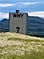# Why is the Standard Error Equal to Sigma Divided by the Square Root of n?Photo Ken Treloar on Unsplash

Every time I teach the Central Limit Theorem, I get questions from students on why we divide the population standard deviation, sigma, by the square root of the sample size to calculate the standard deviation of the sampling distribution which we call the standard error.

Recall that the equation for the standard error is## Dawn Wright

Transwoman, Early TransAdvocate, USAF Academy Grad, Ph.D., Adjunct Professor Statistics, Perpetual Learner